1596040980

# Overview

1. Understand the requirement of feature transformation and training techniques
2. Get to know different feature transformation and scaling techniques including-
• MinMax Scaler
• Standard Scaler
• Power Transformer Scaler
• Unit Vector Scaler/Normalizer

# Introduction

In my machine learning journey, more often than not, I have found that feature preprocessing is a more effective technique in improving my evaluation metric than any other step, like choosing a model algorithm, hyperparameter tuning, etc.

Feature preprocessing is one of the most crucial steps in building a Machine learning model. Too few features and your model won’t have much to learn from. Too many features and we might be feeding unnecessary information to the model. Not only this, but the values in each of the features need to be considered as well.

We know that there are some set rules of dealing with categorical data, as in, encoding them in different ways. However, a large chunk of the process involves dealing with continuous variables. There are various methods of dealing with continuous variables. Some of them include converting them to a normal distribution or converting them to categorical variables, etc.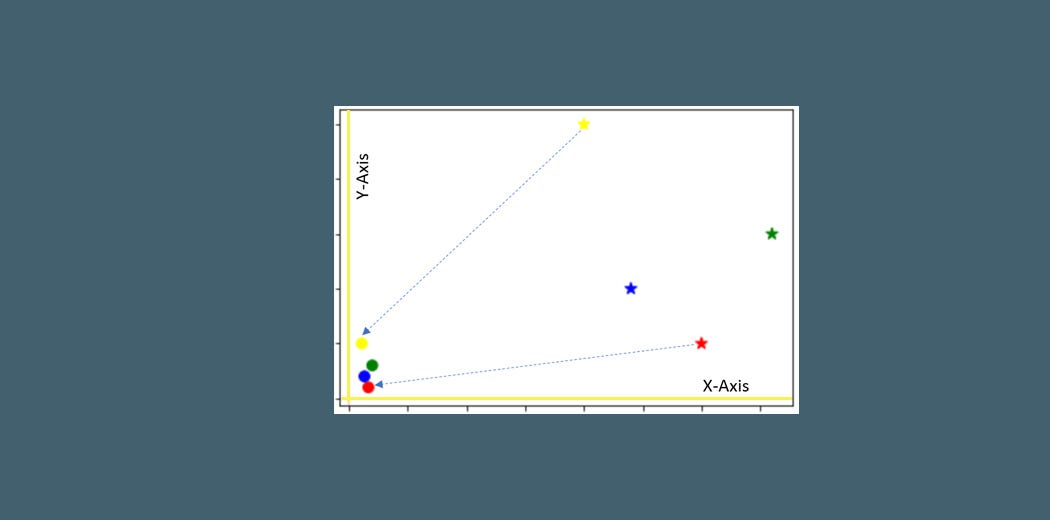There are a couple of go-to techniques I always use regardless of the model I am using, or whether it is a classification task or regression task, or even an unsupervised learning model. These techniques are:

• Feature Transformation and
• Feature Scaling.

_To get started with Data Science and Machine Learning, check out our course — _Applied Machine Learning — Beginner to Professional

1. Why do we need Feature Transformation and Scaling?
2. MinMax Scaler
3. Standard Scaler
4. MaxAbsScaler
5. Robust Scaler
6. Quantile Transformer Scaler
7. Log Transformation
8. Power Transformer Scaler
9. Unit Vector Scaler/Normalizer

## Why do we need Feature Transformation and Scaling?

Oftentimes, we have datasets in which different columns have different units — like one column can be in kilograms, while another column can be in centimeters. Furthermore, we can have columns like income which can range from 20,000 to 100,000, and even more; while an age column which can range from 0 to 100(at the most). Thus, Income is about 1,000 times larger than age.

But how can we be sure that the model treats both these variables equally? When we feed these features to the model as is, there is every chance that the income will influence the result more due to its larger value. But this doesn’t necessarily mean it is more important as a predictor. So, to give importance to both Age, and Income, we need feature scaling.

In most examples of machine learning models, you would have observed either the Standard Scaler or MinMax Scaler. However, the powerful sklearn library offers many other scaling techniques and feature transformations as well, which we can leverage depending on the data we are dealing with. So, what are you waiting for?

Let us explore them one by one with Python code.

We will work with a simple dataframe:

``````import pandas as pd
import numpy as np
import matplotlib.pyplot as plt
%matplotlib inline
df = pd.DataFrame({ 'Income': [15000, 1800, 120000, 10000],
'Age': [25, 18, 42, 51],
'Department': ['HR','Legal','Marketing','Management'] })
``````

Before directly applying any feature transformation or scaling technique, we need to remember the categorical column: Department and first deal with it. This is because we cannot scale non-numeric values.

For that, we 1st create a copy of our dataframe and store the numerical feature names in a list, and their values as well:

``````df_scaled = df.copy() col_names = ['Income', 'Age']
features = df_scaled[col_names]
``````

We will execute this snippet before using a new scaler every time.

## MinMax Scaler

The MinMax scaler is one of the simplest scalers to understand. It just scales all the data between 0 and 1. The formula for calculating the scaled value is-

x_scaled = (x — x_min)/(x_max — x_min)

Thus, a point to note is that it does so for every feature separately. Though (0, 1) is the default range, we can define our range of max and min values as well. How to implement the MinMax scaler?

1 — We will first need to import it

``````from sklearn.preprocessing import MinMaxScaler
scaler = MinMaxScaler()
``````

2 — Apply it on only the values of the features:

``````df_scaled[col_names] = scaler.fit_transform(features.values)
``````

How do the scaled values look like?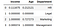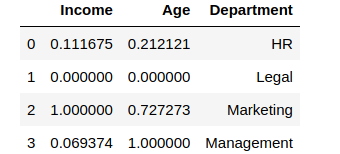You can see how the values were scaled. The minimum value among the columns became 0, and the maximum value was changed to 1, with other values in between. However, suppose we don’t want the income or age to have values like 0. Let us take the range to be (5, 10)

``````from sklearn.preprocessing import MinMaxScaler
scaler = MinMaxScaler(feature_range=(5, 10))

df_scaled[col_names] = scaler.fit_transform(features.values)
df_scaled
``````

This is what the output looks like: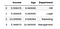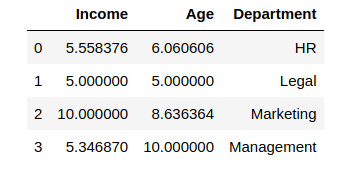Amazing, right? The min-max scaler lets you set the range in which you want the variables to be.

## Standard Scaler

Just like the MinMax Scaler, the Standard Scaler is another popular scaler that is very easy to understand and implement.

For each feature, the Standard Scaler scales the values such that the mean is 0 and the standard deviation is 1(or the variance).

x_scaled = x — mean/std_dev

However, Standard Scaler assumes that the distribution of the variable is normal. Thus, in case, the variables are not normally distributed, we

1. either choose a different scaler
2. or first, convert the variables to a normal distribution and then apply this scaler

Implementing the standard scaler is much similar to implementing a min-max scaler. Just like before, we will first import StandardScaler and then use it to transform our variable.

#feature-engineering #feature-scaling #scikit-learn #deep learning

## Buddha Community1596040980

# Overview

1. Understand the requirement of feature transformation and training techniques
2. Get to know different feature transformation and scaling techniques including-
• MinMax Scaler
• Standard Scaler
• Power Transformer Scaler
• Unit Vector Scaler/Normalizer

# Introduction

In my machine learning journey, more often than not, I have found that feature preprocessing is a more effective technique in improving my evaluation metric than any other step, like choosing a model algorithm, hyperparameter tuning, etc.

Feature preprocessing is one of the most crucial steps in building a Machine learning model. Too few features and your model won’t have much to learn from. Too many features and we might be feeding unnecessary information to the model. Not only this, but the values in each of the features need to be considered as well.

We know that there are some set rules of dealing with categorical data, as in, encoding them in different ways. However, a large chunk of the process involves dealing with continuous variables. There are various methods of dealing with continuous variables. Some of them include converting them to a normal distribution or converting them to categorical variables, etc.There are a couple of go-to techniques I always use regardless of the model I am using, or whether it is a classification task or regression task, or even an unsupervised learning model. These techniques are:

• Feature Transformation and
• Feature Scaling.

_To get started with Data Science and Machine Learning, check out our course — _Applied Machine Learning — Beginner to Professional

1. Why do we need Feature Transformation and Scaling?
2. MinMax Scaler
3. Standard Scaler
4. MaxAbsScaler
5. Robust Scaler
6. Quantile Transformer Scaler
7. Log Transformation
8. Power Transformer Scaler
9. Unit Vector Scaler/Normalizer

## Why do we need Feature Transformation and Scaling?

Oftentimes, we have datasets in which different columns have different units — like one column can be in kilograms, while another column can be in centimeters. Furthermore, we can have columns like income which can range from 20,000 to 100,000, and even more; while an age column which can range from 0 to 100(at the most). Thus, Income is about 1,000 times larger than age.

But how can we be sure that the model treats both these variables equally? When we feed these features to the model as is, there is every chance that the income will influence the result more due to its larger value. But this doesn’t necessarily mean it is more important as a predictor. So, to give importance to both Age, and Income, we need feature scaling.

In most examples of machine learning models, you would have observed either the Standard Scaler or MinMax Scaler. However, the powerful sklearn library offers many other scaling techniques and feature transformations as well, which we can leverage depending on the data we are dealing with. So, what are you waiting for?

Let us explore them one by one with Python code.

We will work with a simple dataframe:

``````import pandas as pd
import numpy as np
import matplotlib.pyplot as plt
%matplotlib inline
df = pd.DataFrame({ 'Income': [15000, 1800, 120000, 10000],
'Age': [25, 18, 42, 51],
'Department': ['HR','Legal','Marketing','Management'] })
``````

Before directly applying any feature transformation or scaling technique, we need to remember the categorical column: Department and first deal with it. This is because we cannot scale non-numeric values.

For that, we 1st create a copy of our dataframe and store the numerical feature names in a list, and their values as well:

``````df_scaled = df.copy() col_names = ['Income', 'Age']
features = df_scaled[col_names]
``````

We will execute this snippet before using a new scaler every time.

## MinMax Scaler

The MinMax scaler is one of the simplest scalers to understand. It just scales all the data between 0 and 1. The formula for calculating the scaled value is-

x_scaled = (x — x_min)/(x_max — x_min)

Thus, a point to note is that it does so for every feature separately. Though (0, 1) is the default range, we can define our range of max and min values as well. How to implement the MinMax scaler?

1 — We will first need to import it

``````from sklearn.preprocessing import MinMaxScaler
scaler = MinMaxScaler()
``````

2 — Apply it on only the values of the features:

``````df_scaled[col_names] = scaler.fit_transform(features.values)
``````

How do the scaled values look like?You can see how the values were scaled. The minimum value among the columns became 0, and the maximum value was changed to 1, with other values in between. However, suppose we don’t want the income or age to have values like 0. Let us take the range to be (5, 10)

``````from sklearn.preprocessing import MinMaxScaler
scaler = MinMaxScaler(feature_range=(5, 10))

df_scaled[col_names] = scaler.fit_transform(features.values)
df_scaled
``````

This is what the output looks like:Amazing, right? The min-max scaler lets you set the range in which you want the variables to be.

## Standard Scaler

Just like the MinMax Scaler, the Standard Scaler is another popular scaler that is very easy to understand and implement.

For each feature, the Standard Scaler scales the values such that the mean is 0 and the standard deviation is 1(or the variance).

x_scaled = x — mean/std_dev

However, Standard Scaler assumes that the distribution of the variable is normal. Thus, in case, the variables are not normally distributed, we

1. either choose a different scaler
2. or first, convert the variables to a normal distribution and then apply this scaler

Implementing the standard scaler is much similar to implementing a min-max scaler. Just like before, we will first import StandardScaler and then use it to transform our variable.

#feature-engineering #feature-scaling #scikit-learn #deep learning1590551696

## Feature Scaling. Why we go for Feature Scaling ?

What is Feature Scaling ?
Feature Scaling is done on the dataset to bring all the different types of data to a Single Format. Done on Independent Variable.
Some Algorithm, uses Euclideam Distance to calculate the target. If the data varies in Magnitude and Units, Distance between the Independent Variables will be more. SO,bring the data in such a way that Independent variables looks same and does not vary much in terms of magnitude.

#standardscalar #scaling-pandas #minmaxscalar #feature-scaling

1624252974

## Digital Transformation Consulting Services & solutions

Compete in this Digital-First world with PixelCrayons’ advanced level digital transformation consulting services. With 16+ years of domain expertise, we have transformed thousands of companies digitally. Our insight-led, unique, and mindful thinking process helps organizations realize Digital Capital from business outcomes.

Let our expert digital transformation consultants partner with you in order to solve even complex business problems at speed and at scale.

Digital transformation company in india

#digital transformation agency #top digital transformation companies in india #digital transformation companies in india #digital transformation services india #digital transformation consulting firms1596716340

## Transformers for Natural Language Processing

It may seem like a long time since the world of natural language processing (NLP) was transformed by the seminal “Attention is All You Need” paper by Vaswani et al., but in fact that was less than 3 years ago. The relative recency of the introduction of transformer architectures and the ubiquity with which they have upended language tasks speaks to the rapid rate of progress in machine learning and artificial intelligence. There’s no better time than now to gain a deep understanding of the inner workings of transformer architectures, especially with transformer models making big inroads into diverse new applications like predicting chemical reactions and reinforcement learning.

Whether you’re an old hand or you’re only paying attention to transformer style architecture for the first time, this article should offer something for you. First, we’ll dive deep into the fundamental concepts used to build the original 2017 Transformer. Then we’ll touch on some of the developments implemented in subsequent transformer models. Where appropriate we’ll point out some limitations and how modern models inheriting ideas from the original Transformer are trying to overcome various shortcomings or improve performance.

## What Do Transformers Do?

Transformers are the current state-of-the-art type of model for dealing with sequences. Perhaps the most prominent application of these models is in text processing tasks, and the most prominent of these is machine translation. In fact, transformers and their conceptual progeny have infiltrated just about every benchmark leaderboard in natural language processing (NLP), from question answering to grammar correction. In many ways transformer architectures are undergoing a surge in development similar to what we saw with convolutional neural networks following the 2012 ImageNet competition, for better and for worse.

#natural language processing #ai artificial intelligence #transformers #transformer architecture #transformer models1603350000

## Feature Scaling Techniques — All You Need To Know

Feature Scaling is a Data Preprocessing step used to normalize the features in the dataset to make sure that all the features lie in a similar range.

## Why Feature Scaling?

Most of the real-life datasets that you will be dealing with will have many features ranging from a wide range of values.

Like if you are working with a machine learning problem in which you need to predict the price of a house, you will be provided with many features like no.of. bedrooms, square feet area of the house, etc…As you can guess, the no. of bedrooms will vary between 1 and 5, but the square feet area will range from 500–2000. This is a huge difference in the range of both features.

Many machine learning algorithms that are using euclidean distance as a metric to calculate the similarities will fail to give a reasonable recognition to the smaller feature, in this case, the number of bedrooms, which in the real case can turn out to be an actually important metric.

Some of the machine learning algorithm, that needs feature scaling for better prediction are Linear Regression, Logistic Regression, KNN, KMeans, Principal Component Analysis, etc.

There are several ways to scale your dataset. I will be discussing on 5 of the most commonly used feature scaling techniques.

1. Absolute Maximum Scaling
2. Min-Max Scaling
3. Normalization
4. Standardization
5. Robust Scaling

Originally Posted on my Website — Let’s Discuss Stuff

#normalization #feature-scaling #machine-learning #statistics #python